# 2 Model Equations

We consider a plasma consisting of three components, namely a cool electron-fluid (at temperature), an inertialess hot electron component with a nonthermal () velocity distribution, and uniformly distributed stationary ions.

The cool electron behavior is governed by the continuity equation,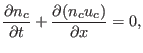(2)

and the momentum equation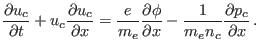(3)

The pressure of the cool electrons is governed by(4)

Here,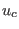andare the number density, the velocity and the pressure of the cool electron fluid,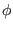is the electrostatic wave potential,the elementary charge, and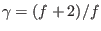denotes the specific heat ratio (fordegrees of freedom). We shall assume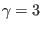(viz.,in 1D) for the adiabatic cool electrons.

We assume the ions to be stationary (immobile), i.e., in a uniform stateconst. (whereis the undisturbed ion density) at all times. In order to obtain an expression for the number density of the hot electrons,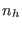, based on thedistribution (1), one may integrate Eq. (1) over the velocity space, to obtain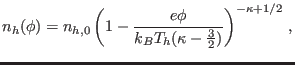(5)

where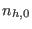and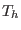are the equilibrium number density and “temperature” of the hot electrons, respectively, andis the spectral index measuring the deviation from thermal equilibrium.

The densities of the (-distributed) hot electrons, the adiabatic cool electrons, and the stationary ions are coupled via Poisson's equation:(6)

where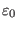is the permittivity constant,andare the number density of hot electrons and ions, respectively.

At equilibrium, the plasma is quasi-neutral, so that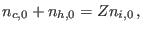(7)

implying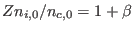, where we have defined the hot-to-cool electron density ratio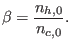(8)

According to Ref. , Landau damping is minimized in the range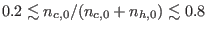, implying. This is our region of interest in what follows, as nonlinear structures will not be sustainable for plasma configurations for which the linear waves are strongly damped.

Scaling by appropriate quantities, we obtain the normalized set of equations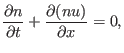(9)(10)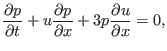(11)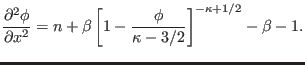(12)

Here,,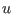and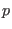denote the cool electron fluid density, velocity and pressure variables normalized with respect to,and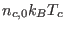, respectively. Time and space were scaled by the plasma period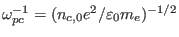and the characteristic length, respectively. Finally,is the wave potential scaled by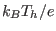. We have defined the temperature ratio of the cool to the hot electrons as(13)

Ashkbiz Danehkar
2018-03-28